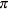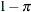MODEL Statement

MODEL response <(response-options)> = <fixed-effects> </ model-options> ;

MODEL events/trials = <fixed-effects> </ model-options> ;

The MODEL statement is required and names the dependent variable and the fixed effects. The fixed-effects determine thematrix of the model (see the section Notation for the Generalized Linear Mixed Model for details). The specification of effects is the same as in the GLM or MIXED procedure. In contrast to PROC GLM, you do not specify random effects in the MODEL statement. However, in contrast to PROC GLM and PROC MIXED, continuous variables on the left and right side of the MODEL statement can be computed through PROC GLIMMIX programming statements.

An intercept is included in the fixed-effects model by default. It can be removed with the NOINT option.

The dependent variable can be specified by using either the response syntax or the events/trials syntax. The events/trials syntax is specific to models for binomial data. A binomial(n,) variable is the sum of n independent Bernoulli trials with event probability. Each Bernoulli trial results in either an event or a nonevent (with probability). You use the events/trials syntax to indicate to the GLIMMIX procedure that the Bernoulli outcomes are grouped. The value of the second variable, trials, gives the number n of Bernoulli trials. The value of the first variable, events, is the number of events out of n. The values of both events and (trialsevents) must be nonnegative and the value of trials must be positive. Observations for which these conditions are not met are excluded from the analysis. If the events/trials syntax is used, the GLIMMIX procedure defaults to the binomial distribution. The response is then the events variable. The trials variable is accounted in model fitting as an additional weight. If you use the response syntax, the procedure defaults to the normal distribution.

There are two sets of options in the MODEL statement. The response-options determine how the GLIMMIX procedure models probabilities for binary and multinomial data. The model-options control other aspects of model formation and inference. Table 41.10 summarizes the options available in the MODEL statement. These are subsequently discussed in detail in alphabetical order by option category.

Table 41.10: MODEL Statement Options

Option

Description

Response Variable Options

Reverses the order of response categories

Specifies the event category in binary models

Specifies the sort order for the response variable

Specifies the reference category in generalized logit models

Model Building

Specifies the response distribution

Excludes fixed-effect intercept from model

Specifies the offset variable for linear predictor

Statistical Computations

ALPHA=Determines the confidence level ()

Requests chi-square tests

Specifies the denominator degrees of freedom (list)

Specifies the method for computing denominator degrees of freedom

Selects the type of hypothesis test

Determines how weights are used

Suppresses centering and scaling ofcolumns during the estimation phase

Specifies a value for the linear predictor

Tunes sensitivity in computing Type III functions

Statistical Output

Displays confidence limits for fixed-effects parameter estimates

Displays the correlation matrix of fixed-effects parameter estimates

Displays the covariance matrix of fixed-effects parameter estimates

Displays the inverse covariance matrix of fixed-effects parameter estimates

E, E1, E2, E3

Displays thematrix coefficients

Adds a row for the intercept to test tables

Displays odds ratios and confidence limits

Displays fixed-effects parameter estimates (and scale parameter in GLM models)

Displays standardized coefficients